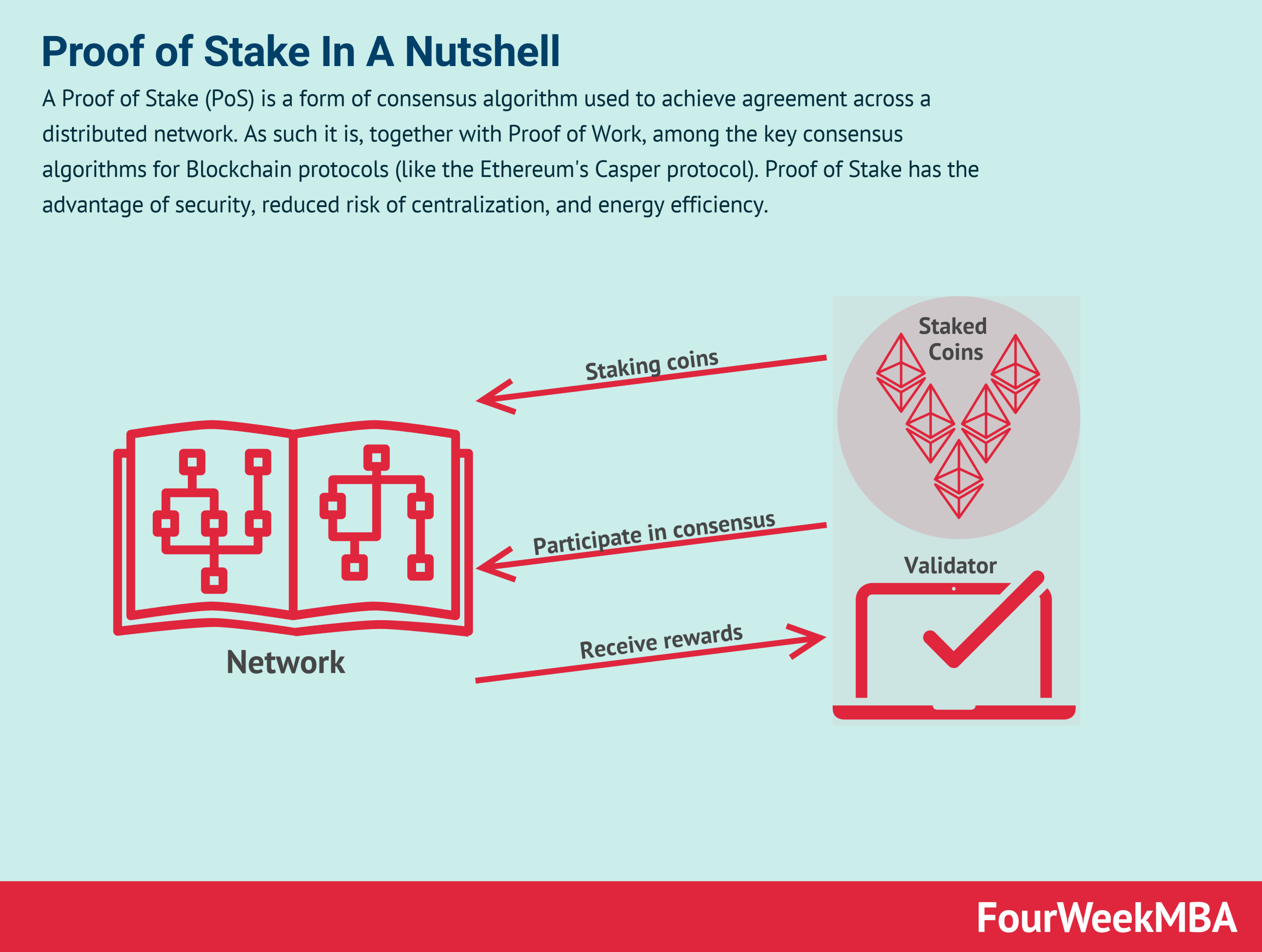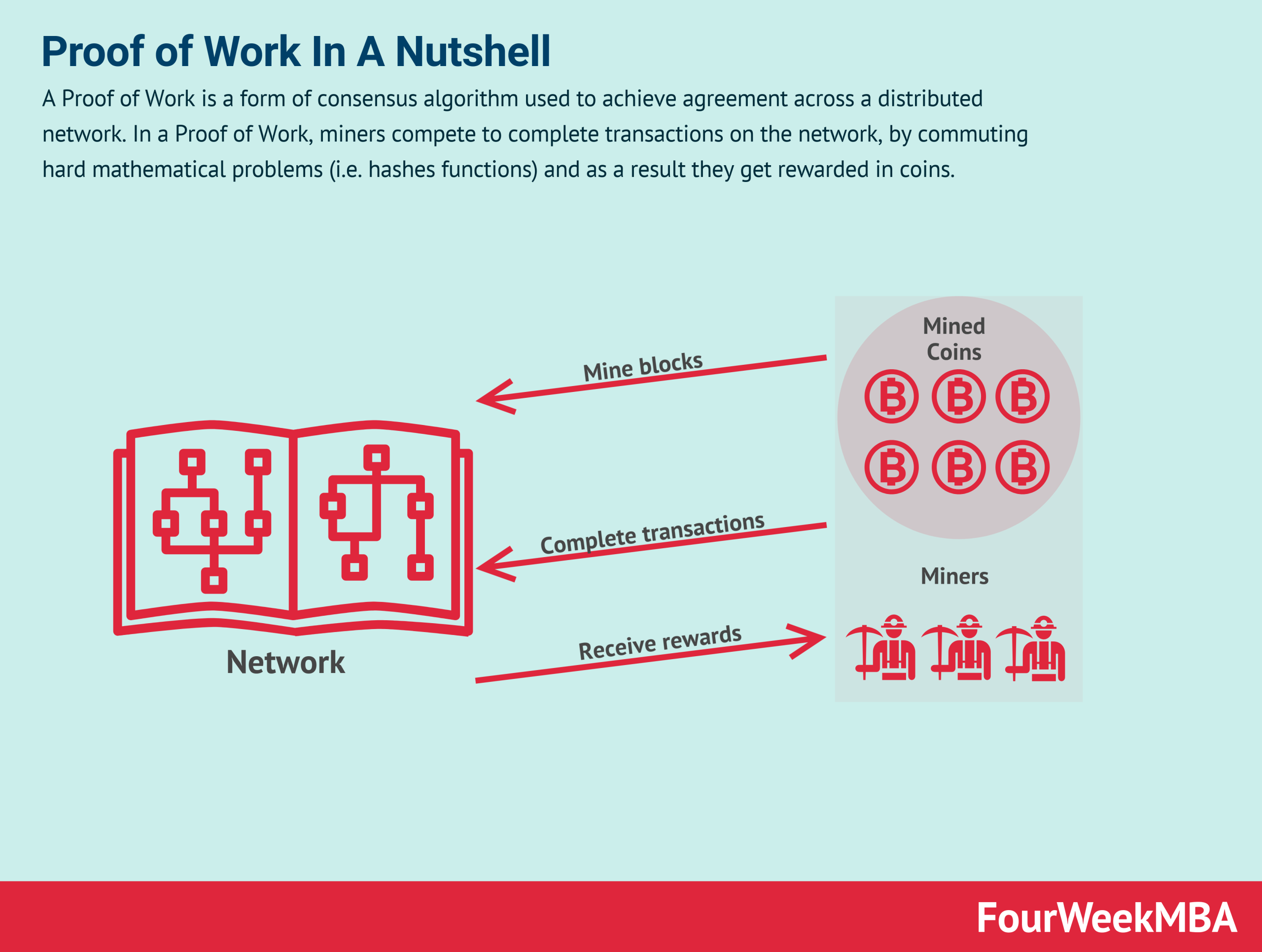# Proof of Work vs. Proof of Stake In A Nutshell

Proof of Work and Proof of Stake are the two primary philosophies and consensus algorithms behind the Blockchain protocols, since the inception of Bitcoin. For instance, Bitcoin still runs through Proof of Work. While other protocols, like Ethereum moved toward Proof of Stake.

### Proof of Stake in a nutshellA Proof of Stake (PoS) is a form of consensus algorithm used to achieve agreement across a distributed network. As such it is, together with Proof of Work, among the key consensus algorithms for Blockchain protocols (like the Ethereum’s Casper protocol). Proof of Stake has the advantage of security, reduced risk of centralization, and energy efficiency.

A Proof of Stake (PoS) is a form of consensus algorithm used to achieve agreement across a distributed network. As such it is, together with Proof of Work, among the key consensus algorithms for Blockchain protocols (like the Ethereum‘s Casper protocol). Proof of Stake has the advantage of security, reduced risk of centralization, and energy efficiency.

### Proof of Work in a nutshellA Proof of Work is a form of consensus algorithm used to achieve agreement across a distributed network. In a Proof of Work, miners compete to complete transactions on the network, by commuting hard mathematical problems (i.e. hashes functions) and as a result they get rewarded in coins.

A Proof of Work is a form of consensus algorithm used to achieve agreement across a distributed network. As such it is, together with Proof of Work, among the key consensus algorithms for Blockchain protocols (like the Ethereum‘s Casper protocol). Proof of Stake has the advantage of security, reduced risk of centralization, and energy efficiency.

## Key takeaways

Consensus algorithms help Blockchain Protocols reach agreement among a distributed network. Among those consensus algorithm there are two main philosophies: Proof of Stake and Proof of Work.

The Proof of Stake determines the consensus based on the stake of each user in the network.

In a Proof of Work, miners compete to complete transactions on the network, by commuting hard mathematical problems (i.e. hashes functions) and as a result they get rewarded in coins.